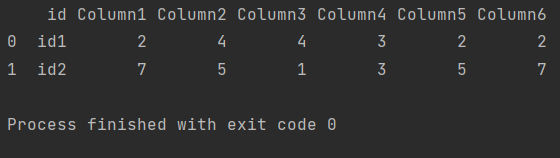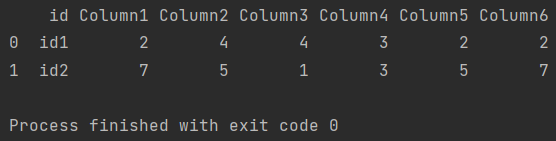Pandas How To Uncategorized How to remove rows with certain values

# How to remove rows with certain values

In this post you will learn how to remove rows with certain values.

In data analysis, you must be prepared to spend most of your time cleaning and preparing data. To help, I’ve prepared a code that will help you delete rows from the dataframe provided that there is specific data in the column.

## How to remove rows with certain values

With this script, I delete rows for which there is a value entered by me in a given column.

```import pandas as pd

my_df = pd.DataFrame({'id':['id1','id2','id4'],
'Column1': ['2', '7', '6'],
'Column2': ['4', '5', '8'],
'Column3': ['4', '1', '9'],
'Column4': ['3', '3', '8'],
'Column5': ['2', '5', '4'],
'Column6': ['2', '7', '3']},)

my_df.drop(index=my_df[my_df['Column2'] == '8'].index, inplace=True)

print(my_df)

```

Pandas found the value I was looking for and deleted the entire row.## How to remove rows with certain substring

With this code, I delete rows provided that the substring I entered is present in the column I specify.

```import pandas as pd

my_df = pd.DataFrame({'id':['id1','id2','id4'],
'Column1': ['2', '7', '6'],
'Column2': ['4', '5', 'foobar'],
'Column3': ['4', '1', '9'],
'Column4': ['3', '3', '8'],
'Column5': ['2', '5', '4'],
'Column6': ['2', '7', '3']},)

my_df.drop(index=my_df[my_df['Column2'].str.contains('foo')].index, inplace=True)

print(my_df)

```

Pandas found the substring I was looking for in a string and deleted the entire line.Thanks to this post, I hope that the preparation of data in the dataframe will be more pleasant and will take less time.

Tags:

## How to drop duplicates based on two columnsHow to drop duplicates based on two columns

In Pandas, you can drop duplicates based on two columns using the drop_duplicates method with the subset parameter. Here is an example: